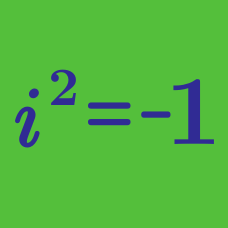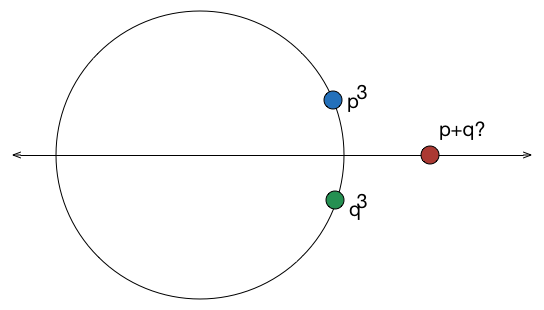Algebra

# Complex Numbers: Level 3 Challenges

If the square of $$x$$ and the square root of $$x$$ are equal to each other, but not equal to $$x$$, then what is the value of $$x^2+x$$?Let $$z=x+iy$$ be a complex number where $$x$$ and $$y$$ are integers. Find the area of the rectangle whose vertices are the roots of the equation $\large z \bar{z}^3+\bar{z}z^3=350.$

Details: $$\bar{z}=x-iy$$ and $$i=\sqrt{-1}$$.

How many complex numbers are the conjugate of their own cube?The complex numbers $$p$$ and $$q$$ satisfy $$p^3=5+i\sqrt{2}$$ and $$q^3=5-i\sqrt{2}$$.

Find the only possible integer value of $$p+q$$.

$\large e ^{ i\theta }e^{ 2i\theta} e^{ 3i\theta }...\large e^{ ni\theta } = 1$

Find the value of $$\theta$$ satisfying the above equation for $$m\in\mathbb{N}$$.

×

Problem Loading...

Note Loading...

Set Loading...